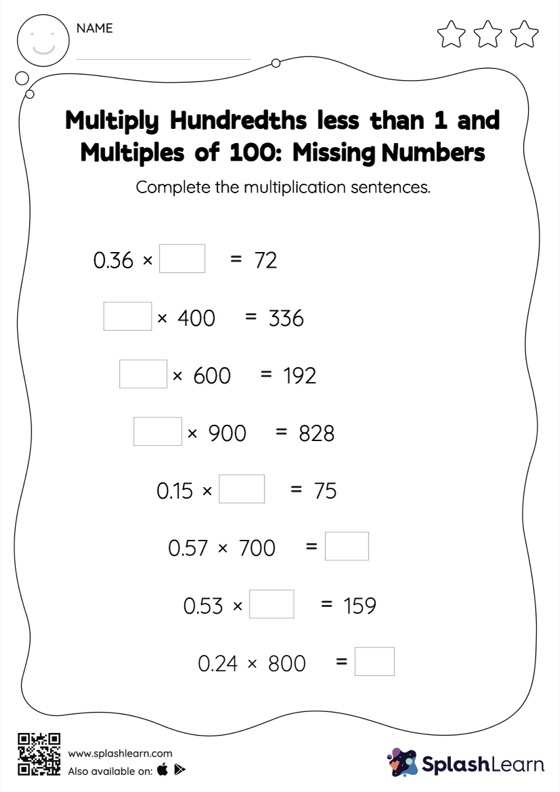# Multiply Hundredths less than 1 and Multiples of 100: Missing Numbers Worksheet

Home > Multiply Hundredths less than 1 and Multiples of 100: Missing NumbersThis multiply hundredths less than 1 and multiples of 100 worksheet consists of tasks to help your young mathematician develop fluency with multiplication. Students understand that when multiplying a decimal by a number ending in zeros, the product will have the decimal point shifted to the right by the same amount as the number of zeros in the multiplier. They practice this concept extensively while finding the missing number in multiply hundredths less than 1 and multiples of 100 worksheet.